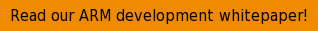Many modern microcontroller devices have more than one memory region, and you may want to locate code or data on fixed memory addresses in any of those memory regions, for various reasons. It is possible to use the linker script in the TrueSTUDIO C/C++ IDE and other GNU/GCC-based ARM Cortex-M development tools to precisely place the code in different memory areas.To do that modify the .ld-linker script file memory regions. Here is an example of a linker script file containing the following memory areas:

`MEMORY`
`{`
`FLASH (rx) : ORIGIN = 0x08000000, LENGTH = 128K`
`RAM (xrw) : ORIGIN = 0x20000000, LENGTH = 16K`
`MEMORY_B1 (rx) : ORIGIN = 0x60000000, LENGTH = 0K`
`}`

Add a new area by editing the linker configuration file. In this example, the IP-Code region is added.

`MEMORY`
`{`
`FLASH (rx)      : ORIGIN = 0x08000000, LENGTH = 64K`
`IP_CODE (x)     : ORIGIN = 0x08010000, LENGTH = 64K`
`RAM (xrw)       : ORIGIN = 0x20000000, LENGTH = 8K`
`MEMORY_B1 (rx)  : ORIGIN = 0x60000000, LENGTH = 0K`
`}`

Place the following code a bit further down in the script, between the .data { ... } and the .bss { ... } section:

`.ip_code :`
`{`
`*(.IP_Code*);`
`} > IP_CODE`

This change tells the linker to place all sections named .IP_Code* into the IP_CODE memory region that is specified to start at target memory address: 0x8010000.

In the C-code, tell the compiler which functions should go to this section by adding __attribute__((section(".IP_Code"))) before the function declaration. Example:

`__attribute__((section(".IP_Code"))) int placed_logic()`
`{`
`/* TODO - Add your application code here */`
`return 1;`
`}`

The placed_logic()-function are now placed in the IP_CODE memory region by the linker.
It is also possible to use the GNU LD linker to position data on individual memory addresses in a similar manner.

The first step in order to place variables at a specified address in memory is to create a new memory region in the linker script (the .ld-file). Take a look at an example of a linker script file containing the following memory areas:

`MEMORY`
`{`
`FLASH (rx)      : ORIGIN = 0x08000000, LENGTH = 128K`
`RAM (xrw)       : ORIGIN = 0x20000000, LENGTH = 16K`
`MEMORY_B1 (rx)  : ORIGIN = 0x60000000, LENGTH = 0K`
`}`

A new memory region should be added by editing the file. In this example add the MYVARS region.

`MEMORY`
`{`
`FLASH (rx)      : ORIGIN = 0x08000000, LENGTH = 64K`
`MYVARS (x)      : ORIGIN = 0x08010000, LENGTH = 64K`
`RAM (xrw)       : ORIGIN = 0x20000000, LENGTH = 8K`
`MEMORY_B1 (rx) : ORIGIN = 0x60000000, LENGTH = 0K`
`}`

Now the memory section should be added. Place the following a bit further down in the script, between the .data { ... } and the .bss { ... } section:

`.myvars :`
`{`
`*(.myvars*);`
`} > MYVARS`

This tells the linker to place all sections named .myvars* from input into the .myvars output section in the MYVARS memory region, which is specified to start at target memory address: 0x8010000. A section can be called almost anything except some predefined names such as data.

Now the variables need to be put in that region.

To be certain the order will stay the same when they are spread over multiple source code files, add each variable to a section of its own. Then map the order of the variables in the linker script.
So for example, the C code could be:

`__attribute__((section(".myvars.VERSION_NUMBER"))) uint32_tVERSION_NUMBER;`
`__attribute__((section(".myvars.CRC"))) uint32_t CRC;`
`__attribute__((section(".myvars.BUILD_ID"))) uint16_t BUILD_ID;`
`__attribute__((section(".myvars.OTHER_VAR"))) uint8_t OTHER_VAR;`

Moreover, then decide the order of the variables in the memory in the linker script by adding the specially named sections like:

`.myvars :`
`{`
`*(.myvars.VERSION_NUMBER)`
`*(.myvars.CRC)`
`*(.myvars.BUILD_ID)`
`*(.myvars*);`
`} > MYVARS`

By using the methods outlined above, it is easy to ensure code or data are linked to specific memory addresses on Cortex-M devices like STM32, Kinetis, LPC, EFM32 or XMC.

If you want to read more on ARM Cortex development and debugging using GNU tools, read this whitepaper: